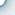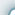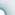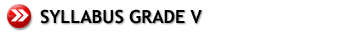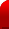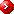Primary Mathematics U.S. Edition 5A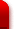Whole NumbersMultiplication and Division by a 2-digit Whole NumberFractions Place Values Millions Approximation and Estimation Multiplying by Tens, Hundreds or Thousands Dividing by Tens, Hundreds or Thousands Order of Operations Word Problems Multiplication Division Fraction and Division Addition and Subtraction of Unlike Fractions Addition and Subtraction of Mixed Numbers Product of a Fraction and a Whole Number Product of Fractions Dividing a Fraction by a Whole Number Word ProblemsArea of Triangle Ratio Angles Finding the Area of a Triangle Finding Ratio Equivalent Ratios Comparing Three Quantities Measuring Angles Finding Unknown Angles Area and Perimeter Rectangles and Squares Composite Figures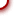Primary Mathematics U.S. Edition 5BDecimalsPercentageAverage Approximation and Estimation Multiplication by Tens, Hundreds or Thousands Division by Tens, Hundreds or Thousands Multiplying by a 2-digit Whole Number Conversion of Measurements Percent Writing Fractions as Percentage Percentage of a Quantity AverageRate Graphs Triangles Rate Line Graphs Sum of Angles of a Triangle Isosceles and Equilateral Triangles Drawing Triangles 4-sided Figures Tessellations Volume Parallelogram, Rhombuses and Trapezoids Drawing Parallelograms and Rhombuses Tiling Patterns Cubes and Cuboids Finding the Volume of a Solid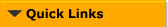cybeRShala Difference Lesson Illustration How It Works Compare Us Request Info Placement Test Tell a friend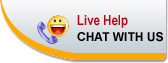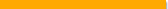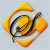Home | FAQ | Tell a Friend | Contact Us | Compare Us | Homeschool Resources | Sitemap cybershala.com Inc. All Rights reserved | Terms of service | Privacy policy